Courses

# Basics of Digital Electronics - 2

## 20 Questions MCQ Test Topicwise Question Bank for Electrical Engineering | Basics of Digital Electronics - 2

Description
This mock test of Basics of Digital Electronics - 2 for Electrical Engineering (EE) helps you for every Electrical Engineering (EE) entrance exam. This contains 20 Multiple Choice Questions for Electrical Engineering (EE) Basics of Digital Electronics - 2 (mcq) to study with solutions a complete question bank. The solved questions answers in this Basics of Digital Electronics - 2 quiz give you a good mix of easy questions and tough questions. Electrical Engineering (EE) students definitely take this Basics of Digital Electronics - 2 exercise for a better result in the exam. You can find other Basics of Digital Electronics - 2 extra questions, long questions & short questions for Electrical Engineering (EE) on EduRev as well by searching above.
QUESTION: 1

### Which of the following statements about digital systems are correct? 1. These are easier to design compared to analog systems. 2. These are highly affected by unwanted electrical signals. 3. Accuracy and precision of digital systems are greater when compared with analog systems. 4. The fabrication of digital ICs is complicated and costly than that of analog ICs. Choose the correct code from the given options:

Solution:

The advantages of digital systems over analog systems are as follows:
1. Easier to design.
2. Less affected by noise (unwanted electrical signals).
3. More accuracy and precision.
4. Simple and economical fabrication into ICs.

QUESTION: 2

### (1010.01)2 - (111.111)2 results into

Solution: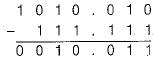In binary system, we take borrow as 2 for subtraction.
Thus, (1010.01)2 - (111.111)2 = (0010.011)2.

QUESTION: 3

### Assertion (A): The advantage of performing subtraction by the complement method is reduction in the hardware. Reason (R): Instead of having separate digital circuits for addition and subtraction, only adding circuits are needed for subtraction by complement method.

Solution:

Both assertion and reason are correct and reason is the correct explanation of assertion because when subtraction is performed using complement method, then instead of subtracting one number from other, the complement of the subtrahend is added to the minued, Thus, subtraction is also performed by adders only as in case of addition. Due to this reason the hardware requirement is reduced while performing subtraction by complement method,

QUESTION: 4

The decimal equivalent of the hexadecimal number (A0F9.0EB)16 is

Solution:

On multiplying the given hexadecimal number with the positional weightage, we get: (A0F9.0EB)16
= (10 x 163) + (0 x 162) + (15 x 161) + (9 x 160) + (0 x 16-1) + (14 x 16-2)+(11 x 16-3)
= 40960 + 0 + 240 + 9 + 0 + 0.0546 + 0.0026
= (41209.0572)10

QUESTION: 5

If 100b = 1610, then the value of b would be equal to

Solution:

On converting 100b to decimal, we have:
1 x b2 + 0 x b1 + 0 x b0 = 16
or, b2 = 16
or, b = 4

QUESTION: 6

Consider the following series with a missing number;
10000,121,100, ?, 24, 22, 20
If in the above series, the same integer is expressed in different number systems, the missing number of the series would be equal to

Solution:
• If we take the first number to be in binary (base 2), its equivalent will be (16)10 i.e. (10000)2 = (16)10.
• The next number is 121; it is equal to (16)10 in base 3 i.e. (121)3 = (16)10
• 100 is equal to (16)10 in base 4 i.e. (100)4 = (16)10.
• (31)s = (16)10
• (24)6 = (16)10
• (22)7 = (16)10
• (20)8 = (16)10

Hence, the missing number is 31 in base 5.

QUESTION: 7

Each of the following arithmetic operations is correct in at least one number system:
(i) 1234 + 5432 = 6666 is valid in any number system with base ≥ 6.
(ii) √41 = 5 is valid in a number system with base 6.
(iii) 302/20 = 12.1 is valid in a number system with base 5.
Out of the above arithmetic operations:

Solution:
• 1234 + 5432 = 6666
i.e.,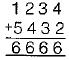Since the largest digit used is 6, this arithmetic operation is valid in any number system with base ≥ 7
• √41 = 5
Let the base be 5.
Then, its decimal equivalent operation is,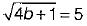or, 4b + 1 = 25 or, b = 6 Thus, the base is 6.
• 302/20 = 12.1
Expressing in decimal, we have:
(3b2 + 2)/2b = b + 2 + 1/b
or, 3b2 + 2 = 2b2 + 4b + 2
or, b2 - 4b = 0 or b = 4
(Neglecting b = 0)
Thus, the base is 4.
Hence, only (ii) is correct.
QUESTION: 8

Consider the following statements:
1. In a self-complementing code, the code word for the 9's complement of N is the 1’s complement of the codeword for N.
2. Each one of the 4-bit BCD codes has 6 illegal states.
3. The Gray code cannot be used for mathematical operations.
4. Excess-3 code can be used for mathematical operations.
Which of the statements given above is/are correct?

Solution:
• A code is said to be self-complementing, if the code word of the 9’s complement of N i.e. of (9-N) can be obtained from the code word of N by interchanging all the 0's and 1s. Thus, statement-1 is correct.
• There are six illegal combinations in 4-bit BCD codes which are 1010, 1011, 1100, 1101, 1110 and 1111 and hence they are not part of the 8421 BCD code system. Thus, statement-2 is also correct.
• The gray code can not be used for mathematical operations because it is non- weighted code. Thus, statement-3 is also correct.
• The excess-3 code is also a non-weighted code, but can be used for mathematical operations because it is a sequential code. Thus, statement-4 is also correct.
QUESTION: 9

The ASCII code is a

Solution:

The ASCII code is a alphanumeric code because it represents alphanumeric information i.e. fetters of the alphabet and decimal numbers as a sequence of 0s and 1s.

QUESTION: 10

Assertion (A): The 2’s complement system has two zeros, a positive 0 (all 0s) and a negative 0 (all 1’s).
Reason (R): The complement of the complement gives the original number

Solution:

The 1’s complement system has a disadvantage of having two zero representation i.e. a positive 0 (all 0s) and a negative 0 (all 1s). But, the 2's complement system has a unique zero.
Hence, assertion is not a correct statement.

QUESTION: 11

Assertion (A): The computer method of division requires successive subtraction.
Reason (R): The computer method of multiplication requires repeated addition.

Solution:
QUESTION: 12

Assertion (A): Gray code is sometimes called reflective code.
Reason (R): The n least significant bits for 2n through (2n +1- 1) are the mirror images for 0 through (2n-1).

Solution:
QUESTION: 13

Which of the following 4-bit binary pairs does not forms a Gray code pair?

Solution:

The Gray code is a cyclic code in which successive code words in this code differ in one bit position only i.e. it is a unit distance code. In options (a), (b) and (d) both the 4-bit binary numbers differ in one bit position only while in option (c) both the 4-bit binary numbers differs in two bit positions as shown below.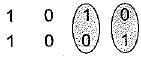Hence, option (c) does not forms a gray code pair of two given 4-bit binary numbers.

QUESTION: 14

Match List-I (Data representation) with List-ll (Range with n-bits) and select the correct answer using the codes given below the lists:
List-1
A. Signed numbers in 1 ’s complement form
B. Signed magnitude
C. Unsigned magnitude
D. Signed numbers in 2’s complement form
List-ll
1. 0 to (2n - 1)
2. -(2n -1) to + (2n -1 - 1)
3. -(2n -1) to (2n -1)
4. -(2n -1 - 1) to + (2n -1 - 1)
Codes:
A B C D
(a) 3 3 1 2
(b) 1 4 3 4
(c) 2 4 1 3
(d) 4 4 1 2

Solution:
QUESTION: 15

A number is represented in 2’s complement as (1101)2. It’s equivalent decimal number is

Solution:

2's complement representation of given number is (1101)2.
Since MSB is 1, therefore the required number must be negative.
1's complement of (1101)2 is (0010)2.
2's complement of 0010 - (0010)2 + (0001)2 = (0011)2.
Thus, the required number = -3 (Decimal equivalent of (0011)2 = 3).

QUESTION: 16

Match List-I with List-ll and select the correct answer using the codes given below the lists:
List-I
A. (345)8
B. (11011.011)2
C. (47)16
List-II
1. (1B.6)16
2. (229)10
3. (107)8
4. (326)10
5. (D8.7)16
Code:
A B C
(a) 2 5 3
(b) 4 3 2
(c) 2 1 3
(d) 4 1 2

Solution:
• (345)8 = (82 x 3) + (81 x 4) + (80 x 5) = (229)10 (Octal to decimal conversion
• (11011.011)2 =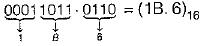(Binary to Hexadecimal conversion)
• (47)16 - (01000111)=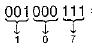= (107)8
(Hexadecimal to Binary and then to Octal Conversion)
QUESTION: 17

Which of the following statements is/are correct in regard to excess-3 code?

Solution:
QUESTION: 18

Multiplication of two binary numbers A and B is 11011. If A = 011, then B is

Solution:

Let,
B = (b3 b2b1b0)2
Now,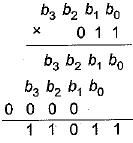Thus,
b0 = 1
b1 + b0 = 1   ∴ b1 = 0
b2 + b1 + 0 =  0   ∴ b2 = 0
b3 + 0 = 1   ∴ b= 1
Thus,
B = (b3b2b1b0)2
= (1001)2

QUESTION: 19

Which of the following statement is not correct in regard to the “GRAY Code”?

Solution:

The Gray code is not a BCD code.

QUESTION: 20

Assertion (A): The “XS-3 code,” also called “Excess-3 code” is a non-weighted BCD code.
Reason (R): In XS-3 code, each binary code word is the corresponding 8421 code word plus 0011(3).

Solution: